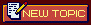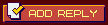## Pete´s Messageboard... No ISO/BIOS requests!

 Homepage Members Register Login Search Old boardAuthorTopics » Book an abo for this threadDanilaZabiakaStatus:Offline
Date registered: 07.05.2020
Post:5
Send MessageCreated on 07.05.2020 - 19:37hmm

Signature
Hehe

DanilaZabiakaStatus:Offline
Date registered: 07.05.2020
Post:5
Send MessageCreated on 07.05.2020 - 19:55So, its me this person who destroyed time. Thank you for chrno cross and cgrno trigger

By the way, can I apply noise shader for different frames?

https://funkyimg.com/i/34HHs.png

https://funkyimg.com/i/34HHt.png

gpuPeteOGL2.slf

[CODE]//patched by Danila Zabiaka - white black noise

#define white 236.0

#define black 20.0

#define noise 10.0

#define offset -15.0

/*

Copyright (C) 2010-2012 cgwg, Themaister and DOLLS

This program is free software; you can redistribute it and/or modify it

Software Foundation; either version 2 of the License, or (at your option)

any later version.

(cgwg gave their consent to have the original version of this shader

http://board.byuu.org/viewtopic.php?p=26075#p26075

"Feel free to distribute my shaders under the GPL. After all, the

barrel distortion code was taken from the Curvature shader, which is

under the GPL."

)

*/

// Comment the next line to disable interpolation in linear gamma (and

// gain speed).

#define LINEAR_PROCESSING

// Enable screen curvature.

//#define CURVATURE

// Enable 3x oversampling of the beam profile

#define OVERSAMPLE

// Use the older, purely gaussian beam profile

//#define USEGAUSSIAN

// Macros.

#define FIX(c) max(abs(c), 1e-5);

#define PI 3.141592653589

#ifdef LINEAR_PROCESSING

# define TEX2D(c) pow(texture2D(OGL2Texture, (c)), vec4(CRTgamma))

#else

# define TEX2D(c) texture2D(OGL2Texture, (c))

#endif

uniform sampler2D OGL2Texture;

uniform vec4 OGL2Param, OGL2Size;

vec2 rubyTextureSize = OGL2Size.xy;

vec2 rubyInputSize = vec2(640.0f, 240.0f);

varying vec2 texCoord;

varying vec2 one;

varying float mod_factor;

varying float CRTgamma;

varying float monitorgamma;

varying vec2 overscan;

varying vec2 aspect;

varying float d;

varying float R;

varying float cornersize;

varying float cornersmooth;

varying vec3 stretch;

varying vec2 sinangle;

varying vec2 cosangle;

float intersect(vec2 xy)

{

float A = dot(xy,xy)+d*d;

float B = 2.0*(R*(dot(xy,sinangle)-d*cosangle.x*cosangle.y)-d*d);

float C = d*d + 2.0*R*d*cosangle.x*cosangle.y;

return (-B-sqrt(B*B-4.0*A*C))/(2.0*A);

}

vec2 bkwtrans(vec2 xy)

{

float c = intersect(xy);

vec2 point = vec2(c)*xy;

point -= vec2(-R)*sinangle;

point /= vec2(R);

vec2 tang = sinangle/cosangle;

vec2 poc = point/cosangle;

float A = dot(tang,tang)+1.0;

float B = -2.0*dot(poc,tang);

float C = dot(poc,poc)-1.0;

float a = (-B+sqrt(B*B-4.0*A*C))/(2.0*A);

vec2 uv = (point-a*sinangle)/cosangle;

float r = FIX(R*acos(a));

return uv*r/sin(r/R);

}

vec2 transform(vec2 coord)

{

coord *= rubyTextureSize / rubyInputSize;

coord = (coord-vec2(0.5))*aspect*stretch.z+stretch.xy;

return (bkwtrans(coord)/overscan/aspect+vec2(0.5)) * rubyInputSize / rubyTextureSize;

}

float corner(vec2 coord)

{

coord *= rubyTextureSize / rubyInputSize;

coord = (coord - vec2(0.5)) * overscan + vec2(0.5);

coord = min(coord, vec2(1.0)-coord) * aspect;

vec2 cdist = vec2(cornersize);

coord = (cdist - min(coord,cdist));

float dist = sqrt(dot(coord,coord));

return clamp((cdist.x-dist)*cornersmooth,0.0, 1.0);

}

// Calculate the influence of a scanline on the current pixel.

//

// 'distance' is the distance in texture coordinates from the current

// pixel to the scanline in question.

// 'color' is the colour of the scanline at the horizontal location of

// the current pixel.

vec4 scanlineWeights(float distance, vec4 color)

{

// "wid" controls the width of the scanline beam, for each RGB

// channel The "weights" lines basically specify the formula

// that gives you the profile of the beam, i.e. the intensity as

// a function of distance from the vertical center of the

// scanline. In this case, it is gaussian if width=2, and

// becomes nongaussian for larger widths. Ideally this should

// be normalized so that the integral across the beam is

// independent of its width. That is, for a narrower beam

// "weights" should have a higher peak at the center of the

// scanline than for a wider beam.

#ifdef USEGAUSSIAN

vec4 wid = 0.3 + 0.1 * pow(color, vec4(3.0));

vec4 weights = vec4(distance / wid);

return 0.4 * exp(-weights * weights) / wid;

#else

vec4 wid = 2.0 + 2.0 * pow(color, vec4(4.0));

vec4 weights = vec4(distance / 0.3);

return 1.4 * exp(-pow(weights * inversesqrt(0.5 * wid), wid)) / (0.6 + 0.2 * wid);

#endif

}

float pseudoNoise(vec2 co)

{

return fract(sin(dot(co.xy ,vec2(12.9898,78.233))) * 43758.5453)*fract(sin(dot(co.xy ,vec2(12.9898,78.233)*2.0)) * 43758.5453); //pseudo random number generator

}

void main()

{

// Here's a helpful diagram to keep in mind while trying to

// understand the code:

//

// | | | | |

// -------------------------------

// | | | | |

// | 01 | 11 | 21 | 31 | <-- current scanline

// | | @ | | |

// -------------------------------

// | | | | |

// | 02 | 12 | 22 | 32 | <-- next scanline

// | | | | |

// -------------------------------

// | | | | |

//

// Each character-cell represents a pixel on the output

// surface, "@" represents the current pixel (always somewhere

// in the bottom half of the current scan-line, or the top-half

// of the next scanline). The grid of lines represents the

// edges of the texels of the underlying texture.

// Texture coordinates of the texel containing the active pixel.

#ifdef CURVATURE

vec2 xy = transform(texCoord);

#else

vec2 xy = texCoord;

#endif

float cval = corner(xy);

// Of all the pixels that are mapped onto the texel we are

// currently rendering, which pixel are we currently rendering?

vec2 ratio_scale = xy * rubyTextureSize - vec2(0.5);

#ifdef OVERSAMPLE

float filter = fwidth(ratio_scale.y);

#endif

vec2 uv_ratio = fract(ratio_scale);

// Snap to the center of the underlying texel.

xy = (floor(ratio_scale) + vec2(0.5)) / rubyTextureSize;

// Calculate Lanczos scaling coefficients describing the effect

// of various neighbour texels in a scanline on the current

// pixel.

vec4 coeffs = PI * vec4(1.0 + uv_ratio.x, uv_ratio.x, 1.0 - uv_ratio.x, 2.0 - uv_ratio.x);

// Prevent division by zero.

coeffs = FIX(coeffs);

// Lanczos2 kernel.

coeffs = 2.0 * sin(coeffs) * sin(coeffs / 2.0) / (coeffs * coeffs);

// Normalize.

coeffs /= dot(coeffs, vec4(1.0));

// Calculate the effective colour of the current and next

// scanlines at the horizontal location of the current pixel,

// using the Lanczos coefficients above.

vec4 col = clamp(mat4(

TEX2D(xy + vec2(-one.x, 0.0)),

TEX2D(xy),

TEX2D(xy + vec2(one.x, 0.0)),

TEX2D(xy + vec2(2.0 * one.x, 0.0))) * coeffs,

0.0, 1.0);

vec4 col2 = clamp(mat4(

TEX2D(xy + vec2(-one.x, one.y)),

TEX2D(xy + vec2(0.0, one.y)),

TEX2D(xy + one),

TEX2D(xy + vec2(2.0 * one.x, one.y))) * coeffs,

0.0, 1.0);

#ifndef LINEAR_PROCESSING

col = pow(col , vec4(CRTgamma));

col2 = pow(col2, vec4(CRTgamma));

#endif

// Calculate the influence of the current and next scanlines on

// the current pixel.

vec4 weights = scanlineWeights(uv_ratio.y, col);

vec4 weights2 = scanlineWeights(1.0 - uv_ratio.y, col2);

#ifdef OVERSAMPLE

uv_ratio.y =uv_ratio.y+1.0/3.0*filter;

weights = (weights+scanlineWeights(uv_ratio.y, col))/3.0;

weights2=(weights2+scanlineWeights(abs(1.0-uv_ratio.y), col2))/3.0;

uv_ratio.y =uv_ratio.y-2.0/3.0*filter;

weights=weights+scanlineWeights(abs(uv_ratio.y), col)/3.0;

weights2=weights2+scanlineWeights(abs(1.0-uv_ratio.y), col2)/3.0;

#endif

vec3 mul_res = (col * weights + col2 * weights2).rgb * vec3(cval);

// Output pixels are alternately tinted green and magenta.

vec3(1.0, 0.7, 1.0),

vec3(0.7, 1.0, 0.7),

floor(mod(mod_factor, 2.0))

);

// Convert the image gamma for display on our output device.

mul_res = pow(mul_res, vec3(1.0 / monitorgamma))*white/255.0+black/255.0-mul_res*black/255.0+(pseudoNoise(texCoord)+offset/100.0)*noise/100.0;

// Color the texel.

gl_FragColor = vec4(mul_res, 1.0);

}[/CODE]

gpuPeteOGL2.slv

[CODE] varying float CRTgamma;

varying float monitorgamma;

varying vec2 overscan;

varying vec2 aspect;

varying float d;

varying float R;

varying float cornersize;

varying float cornersmooth;

varying vec3 stretch;

varying vec2 sinangle;

varying vec2 cosangle;

uniform vec4 OGL2Param, OGL2Size;

vec2 rubyTextureSize = OGL2Size.xy;

vec2 rubyInputSize = vec2(640.0f, 240.0f);

vec2 rubyOutputSize = vec2(1280.0f, 960.0f);

varying vec2 texCoord;

varying vec2 one;

varying float mod_factor;

#define FIX(c) max(abs(c), 1e-5);

float intersect(vec2 xy)

{

float A = dot(xy,xy)+d*d;

float B = 2.0*(R*(dot(xy,sinangle)-d*cosangle.x*cosangle.y)-d*d);

float C = d*d + 2.0*R*d*cosangle.x*cosangle.y;

return (-B-sqrt(B*B-4.0*A*C))/(2.0*A);

}

vec2 bkwtrans(vec2 xy)

{

float c = intersect(xy);

vec2 point = vec2(c)*xy;

point -= vec2(-R)*sinangle;

point /= vec2(R);

vec2 tang = sinangle/cosangle;

vec2 poc = point/cosangle;

float A = dot(tang,tang)+1.0;

float B = -2.0*dot(poc,tang);

float C = dot(poc,poc)-1.0;

float a = (-B+sqrt(B*B-4.0*A*C))/(2.0*A);

vec2 uv = (point-a*sinangle)/cosangle;

float r = R*acos(a);

return uv*r/sin(r/R);

}

vec2 fwtrans(vec2 uv)

{

float r = FIX(sqrt(dot(uv,uv)));

uv *= sin(r/R)/r;

float x = 1.0-cos(r/R);

float D = d/R + x*cosangle.x*cosangle.y+dot(uv,sinangle);

return d*(uv*cosangle-x*sinangle)/D;

}

vec3 maxscale()

{

vec2 c = bkwtrans(-R * sinangle / (1.0 + R/d*cosangle.x*cosangle.y));

vec2 a = vec2(0.5,0.5)*aspect;

vec2 lo = vec2(fwtrans(vec2(-a.x,c.y)).x,

fwtrans(vec2(c.x,-a.y)).y)/aspect;

vec2 hi = vec2(fwtrans(vec2(+a.x,c.y)).x,

fwtrans(vec2(c.x,+a.y)).y)/aspect;

return vec3((hi+lo)*aspect*0.5,max(hi.x-lo.x,hi.y-lo.y));

}

void main()

{

// START of parameters

// gamma of simulated CRT

CRTgamma = 2.4;

// gamma of display monitor (typically 2.2 is correct)

monitorgamma = 2.2;

// overscan (e.g. 1.02 for 2% overscan)

overscan = vec2(0.00,0.00);

// aspect ratio

aspect = vec2(1.0, 0.75);

// lengths are measured in units of (approximately) the width

// of the monitor simulated distance from viewer to monitor

d = 2.0;

R = 1.5;

// (behavior might be a bit wrong if both components are

// nonzero)

const vec2 angle = vec2(0.0,-0.15);

// size of curved corners

cornersize = 0.001;

// border smoothness parameter

// decrease if borders are too aliased

cornersmooth = 1000.0;

// END of parameters

// Do the standard vertex processing.

gl_Position = gl_ModelViewProjectionMatrix * gl_Vertex;

// Precalculate a bunch of useful values we'll need in the fragment

sinangle = sin(angle);

cosangle = cos(angle);

stretch = maxscale();

// Texture coords.

texCoord = gl_MultiTexCoord0.xy;

// The size of one texel, in texture-coordinates.

one = 1.0 / rubyTextureSize;

// Resulting X pixel-coordinate of the pixel we're drawing.

mod_factor = texCoord.x * rubyTextureSize.x * rubyOutputSize.x / rubyInputSize.x;

}[/CODE]

[Dieser Beitrag wurde am 07.05.2020 - 20:00 von DanilaZabiaka aktualisiert]

Signature
Hehe

 Similarly threads: Topics Created by Replies Boardname congratulations Katalinka 6 hockus Congratulations! StarlightDestiny 6 war_chronicle Congratulations! MastaMack 8 553crew Congratulations redangel 2 regman Congratulations! Gunslinger 3 birteff